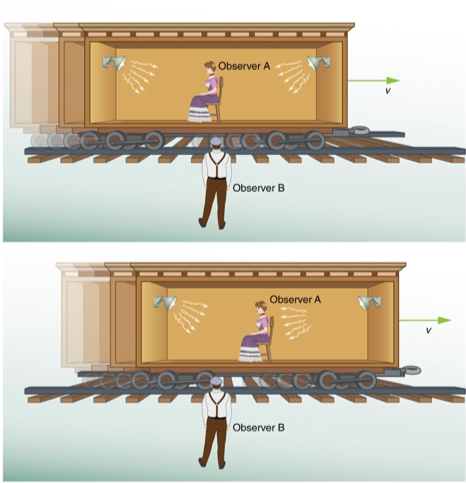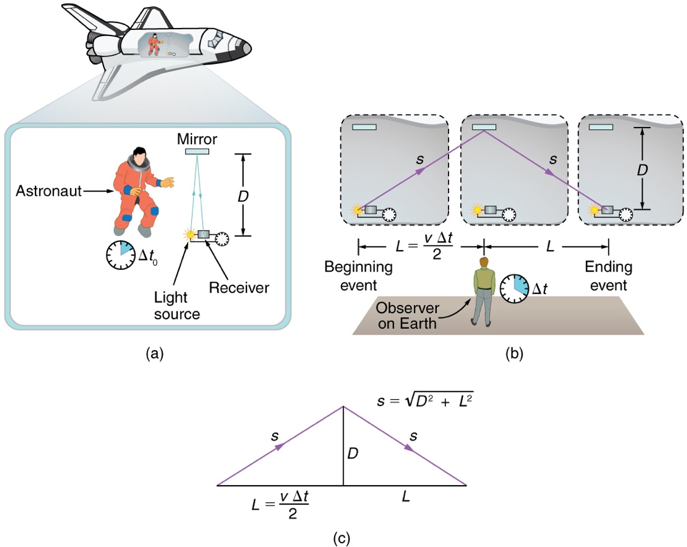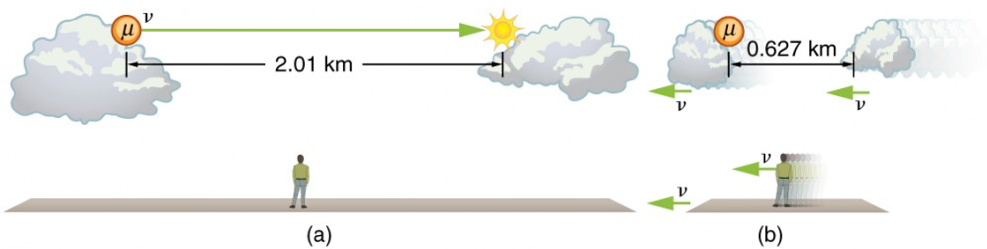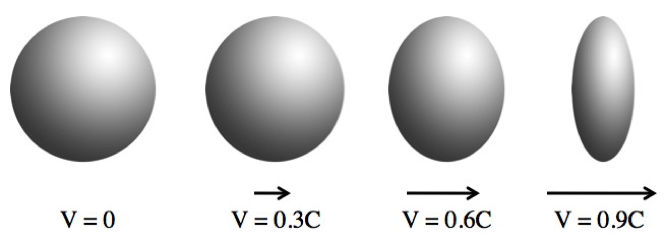# M7-S11: Time Dilation and Length Contraction

• ### investigate the evidence, from Einstein’s thought experiments and subsequent experimental validation, for time dilation t=t_o/sqrt((1-v^2/c^2)) and length contraction l=l_osqrt((1-v^2/c^2)), and analyse quantitatively situations in which these are observed, for example:

• observations of cosmic-origin muons at the Earth’s surface
• atomic clocks (Hafele–Keating experiment)
• evidence from particle accelerators
• evidence from cosmological studies

In this section, we will learn about the ﻿consequences ﻿of special relativity. Time dilation and length contraction are not postulates but the result of the constant nature of light, as proposed by Einstein.

### Simultaneity

• The concept of simultaneity – occurrence of simultaneous events is not absolute. This means two simultaneous events may be so for one observer, they may not occur at the same time for another.
• A simple thought experiment can be used to clarify this understandingFigure 1: Thought experiment demonstrating the effect of special relativity on simultaneity. Credit to Lumen Learning.

Observer A is sitting on a train carriage moving at a substantially fast velocity v. Two sources of light are located at the front and back end of the carriage respectively, equidistant from observer A. When they are turned on simultaneous, observer A receives both stimuli at the same time as the distance between the source and observer remains the same.

Observer B, however, does not observer the sources of light simultaneously. This is because during the time light travels from the source to observer B, the carriage would have displaced by a certain distance. This displacement causes the distance between sources of light to observer B to become different. By Einstein’s second postulate of special relativity (speed of light is constant), the time taken for light to travel a longer distance becomes longer.

### Time Dilation

• The non-absolute nature of simultaneity gives rise to time dilation (one of the three relativistic consequences of the constancy of light)
• The understanding of time dilation can be consolidated and quantiatively analysed using another thought experiment.Figure 2: Thought experiment demonstrating the effect of special relativity on time. Time becomes dilated (longer) when an object is travelling at relativistic speeds as measured by a stationary observer. Credit to Lumen Learning.

Suppose an astronaut wants to measure the time taken for a beam of light to travel back and forth the width (D) of the spaceship, by reflecting off a mirror. The time taken (t0) would be the total distance divided by the velocity of light:

t_o=(2D)/c

However, to an observer outside the spacecraft, the distance travelled by light is longer. To calculate this distance, we need to use Pythagoras’ theorem to obtain:

s=sqrt(D^2+((vt)/2)^2)

Since the distance travelled observed by the person outside the spacecraft is 2s, the time taken for light to travel to and from the mirror in the cabin is:

t=2s/c

By substituting with the expression we derived previously:

t=(2sqrt(D^2+((vt)/2)^2))/c
Square both sides:
t^2=(4(D^2+((vt)/2)^2))/c^2
Simplify:
t^2=(4D^2)/c^2+(v^2*t^2)/c^2

Since the time (t0) taken by light observed by the astronaut inside the spacecraft is:

t_0=(2D)/c

Therefore by substituting:

t^2=t_o^2+(v^2*t^2)/c^2

Rearrange:

t^2-(v^2*t^2)/c^2=t_o^2

Factorise:

t^2(1-v^2/c^2)=t_o^2

Make t2 the subject of equation:

t^2=t_o^2(1-v^2/c^2)

Finally, square root both sides of equation:

t=t_o/sqrt(1-v^2/c^2)

• where t is the time observed by an observer with relative motion to the event being observed. E.g. person outside the spacecraft.
• t0 is the proper time observed by an observer at rest relative to the event being observed. E.g. astronaut moving at the same velocity as the spacecraft.

### Length Contraction

Distance depends on the observer’s relative motion. Since distance is the product of time and speed, shorter time entails a shorter distance covered.The velocity of a particular object relative to an observer at rest is the proper length divided by the dilated time. Proper length L0 is the distance between two points measured by an observer who is at rest relative to both of the points.

v=l_o/t

As such, for an observer who is at rest relative to the moving object (moving at the same speed), velocity is defined as:

v=l/t_o

In this case, the relative velocity in both reference frames are equal:

l_o/t=l/t_o

Make l the subject of equation:

l=l_o(t_o/t)

Since:

t_o/t=sqrt((1-v^2/c^2))

By substitution:

l=l_osqrt((1-v^2/c^2))

• where is the length measured by an observer moving at the same speed as the object travelling the observed length/distance.
• lo is the length measured by an observed at rest relative to the start and end points of the observed length/distance

• An object travelling a high-speed experiences length contraction effect in the direction of its propagation. In other words, its length does not contract in all dimension, only the dimension that is parallel to its direction of travel. It is important to note that length contraction is only observable for an observer at rest relative to the points governing the observed distance or length.### Evidence for time dilation

• Half-life of muons are longer when observed on Earth

Muons are cosmic ray particles found in Earth’s atmosphere. Their short half-life prevented them from reaching Earth from the atmosphere as they decay before they cover the required distance. However, muons are commonly detected on the surface of Earth.

Their half-life at rest (t0) is about 1.5 µs. When they are in motion near the speed of light, their half-life is increased depending on their velocity. This dilated half-life as observed by an Earth-bound observer allows muons to reach the surface of Earth before decaying.

• Atomic clocks verified the effect of time dilation

In 1971, Joseph Hafele and Richard Keating experimented time dilation using three caesium-beam atomic clocks. After flying one eastward and another westward around the world on an aircraft twice, the elapsed time was found to be different on the clocks.

Specifically, the atomic clock, flown eastward, moved slower (as observed from an Earth-bound observer) and consequently lost time. In contrast, the atomic which flew westward, moved faster and gained time.

Evidence for length contraction

Compared to time dilation, the effect of length contraction is difficult to experimentally investigate primarily because either the change in length is so substantially small or the contracted length is vanishingly small.

• Particle accelerators

Experiments involving particle accelerators must account for both length contraction and time dilation. Atoms, ions and sub-atomic particles, such as protons, neutrons and electrons, all undergo length contraction when they are accelerated to high speeds.

Their lengths cannot be directly measured but can be inferring through scattering and diffraction (Moving particles exhibit wave-like properties) experiments. For example, a neutron is commonly used to investigate the structure of new materials; a neutron at relativistic velocity experiences length contraction and may become small enough to pass through lattice structure of a particular material.

However, it is important to note that the length contraction only takes place when their speed becomes constant, not during acceleration. This is because length contraction is a consequence of special relativity which only applies in inertial reference frames.

• Doppler’s Effect

The wavelength of radiation, and light, becomes longer (red-shift) when the source is moving away from us whereas it becomes shorter (blue-shift) when the source is moving towards Earth. This change in length is observable because wavelength is already a small measurement.

Practice Question 1

Suppose a cosmic ray colliding with a nucleus in the Earth’s upper atmosphere produces a muon that has a velocity = 0.950c. The muon then travels at constant velocity and lives 1.52 μs as measured in the muon’s frame of reference. (You can imagine this as the muon’s internal clock.)

How long does the muon live as measured by an Earth-bound observer?

Practice Question 2

A particle is traveling through the Earth’s atmosphere at a speed of 0.750 c. To an Earth-bound observer, the distance it travels is 2.50 km. How far does the particle travel in the particle’s frame of reference?

Practice Question 3

Suppose an astronaut is moving relative to the Earth at a significant fraction of the speed of light.

(a) Does he observe the rate of his clocks to have slowed?

(b) What change in the rate of Earth-bound clocks does he see?

(c) Does his ship seem to him to shorten?

(d) What about the distance between stars that lie on lines parallel to his motion?

(e) Do he and an Earth-bound observer agree on his velocity relative to the Earth?

Previous section: Postulates of Special Relativity

Next section: Relativistic Momentum and Energy-Mass Equivalence